# Graphical Analysis – Page 2

## Bar Charts: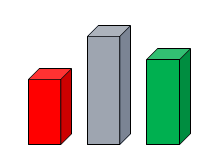Bar charts are ways of displaying frequency of occurrence of attribute data. They focus on the absolute value of the data while a pie chart focuses on the relative value of the data. The bar height indicates the number of times a particular characteristic was observed. The bars on the chart may be arranged in any order and are presented either horizontally or vertically to show comparisons among categories. When a bar chart presents the categories in descending order of frequency, this is called a Pareto Chart.

Some bar charts display bars clustered in groups, and these are called Grouped Bar Charts. Other bar charts stack bars of each group on top of each other to show the cumulative effect, and these are called Stacked Bar Charts. A 100% Staked Bar Chart is used for comparing proportions of categorical data and are useful for demonstrating the difference in proportion between categories.

The following is an example of a grouped bar chart which displays the number of occupied beds in a hospital in two consecutive years.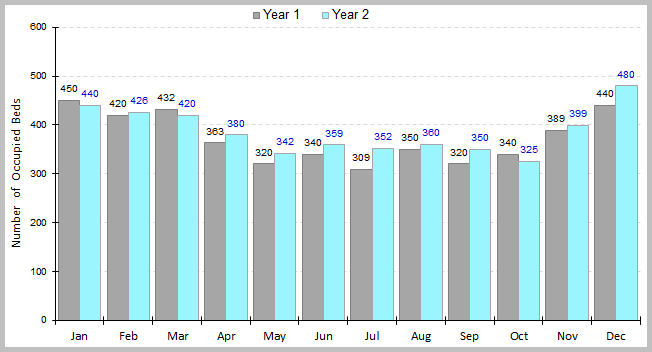The above chart shows the results of a data set that belongs to Minitab Inc.
The following is the same example as above except that it uses a stacked bar chart to display the number of occupied beds in a hospital in two consecutive years.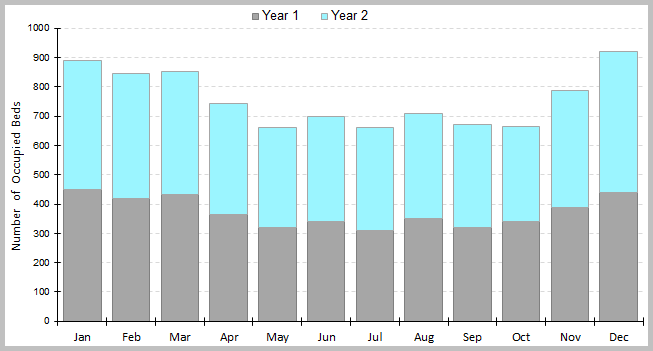The above chart shows the results of a data set that belongs to Minitab Inc.

## Dotplots: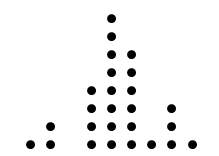A Dotplot is a graphical representation of data using dots plotted on a simple scale. It is a form of frequency distribution that is suitable for displaying small to moderate data sets when the values are not spread out very much. The X-axis in a dotplot is divided into many small intervals called bins, and data values falling within each bin are represented by dots (one or more dots per data point). The end result is a set of vertical lines of evenly-spaced dots.

A dotplot is generally used when the data is discrete, however, it can also be used to present continuous data. It shows where the data are clustered, where the gaps are located and can help identify outliers. Dotplots are also useful for comparing distributions in terms of their shape, location and spread.

The following is a dotplot that displays the number of complaints made by customers in a given period of time (count data).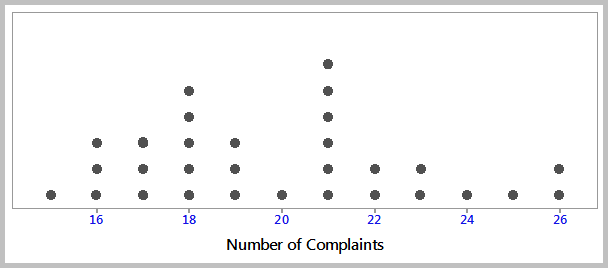The following is an example of a dotplot showing the GPA’s of all students in a business college (continuous variable). Note that each dot represents up to 4 observations.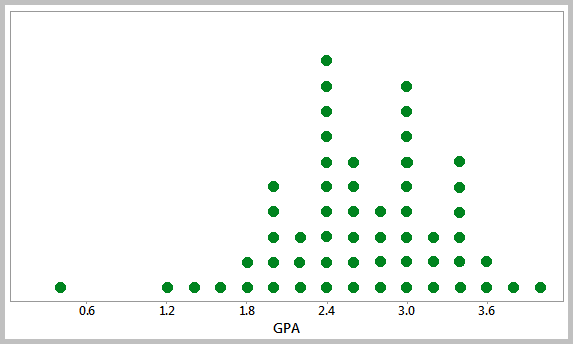In the following example, a dotplot is created to compare the teachers who had been on sick leave between two types of schools during a given period of time.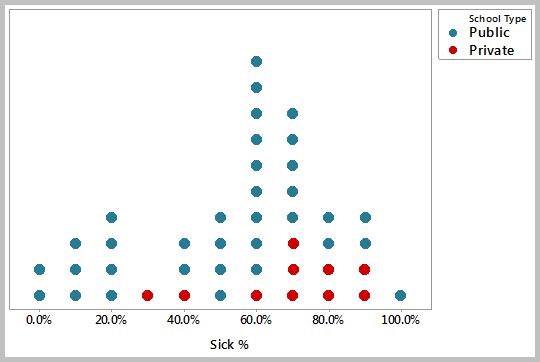The above chart shows the results of a data set that belongs to Minitab Inc.